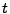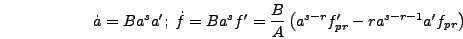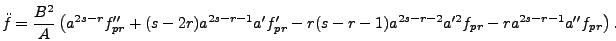Next: Selecting the Rescaling Variables Up: Field Equations and Coordinate Previous: Field Equations and Coordinate

## Field Equations

The equation of motion for a scalar field in an expanding universe is(6.1)

To begin with we will assume a general form for the variable rescalings(6.2)

The position coordinates shouldn't be modified by any powers of the scale factor so that the same comoving wavelengths stay in the box throughout the run. In principle the coefficientcould be different forandbut it is simpler and more useful to keep them the same, thus giving an initial sound speed of one. Below we will derive expressions for these rescaling coordinates in terms of parameters in the potential of the model being solved. To express the field equation in program variables note that(6.3)(6.4)

Finally define a new potential(6.5)

(The potential rescaling is chosen to be consistent with an energy rescaling that gives.) Then putting in all rescalings the equation of motion becomes(6.6)Next: Selecting the Rescaling Variables Up: Field Equations and Coordinate Previous: Field Equations and Coordinate